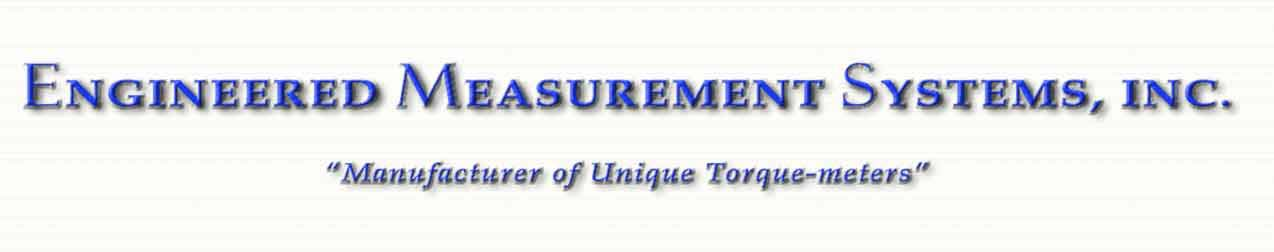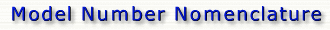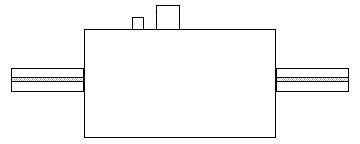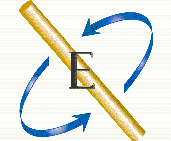B A S E - C A P - S P C - E M S

 Base Models Capacity Values Specification Codes Factory Codes B: Signal Transmission Type C: Full Scale Capacity S: Excitation Frequency E:    Internal A: Housing Style A: Overload Capability P: Error Specification M:  Processing S: Torque Transmission Interface P: Output Type C: Maximum RPM S:       Only Example: Disclaimer

" Disclaimer "
 All information provided in this web site is believed to be accurate and reliable. However, Engineered Measurement Systems assumes no responsibility for the use of this information. Product information is subject to change without notice. If you believe that information obtained from this EMS web site is inaccurate or out-of-date, or if you encounter technical problems, please notify the EMS webmaster.

" Model Number Nomenclature Example "

 5 1 1 5 - F 5 2 - 2 A 4 - E M S

 A base model "5115" is an inline rotating torque meter with non-contact Rotary Transformer Signal Transmission.  It has a Floating Aluminum Housing and Double Male/ Double Key Shaft and is in the 2,000 to 10,000 in.-lbs. capacity range. "F52" designates a full scale capacity of 5000 in. lbs., with an overload capability of 500% of full scale or 25,000 in. lbs. and a full scale output of 2mV/V. "2A4" specifies an excitation frequency of 3 kHz, Non-Repeatability: <0.02% of rated output, Non-linearity: <0.05% of rated output, Hysteresis: <0.05% of rated output, Zero balance: ±2% of rated output, Bridge resistance: 350 ohms nominal, Useable temperature range: -65 to+170° F, Temperature effect on output: ±0.001% / ° F  of rated output Temperature effect on zero balance ±0.001% / ° F of rated output, Excitation voltage 3 to 10 AC  RMS, Bridge current at 5 VAC 50  ma, and Insulation resistance: >5000 mega ohms bridge to case.BASE MODELS

 B A S E - C A P - S P C - E M S

" Signal Transmission Type "

 1 = Slip Ring 2 = Inductive 3 = FM Telemetry 4 = Infra-red 5 = Rotary Transformer 6 = Optical Analog 7 = Optical Digital 8 = Non-Rotating 9 = Universal

BASE MODELS

 B A S E - C A P - S P C - E M S

" Housing Style "

 0 = No Housing  Covers Over Sensor 1 = Floating Aluminum 2 = Floating Steel 3 = Floating Beryllium 4 = Floating / Optional Foot Mount 5 = Optional Foot Mount Installed 6 = Integral Foot Mount / 2 Bearings / Mild Steel 7 = Integral Foot Mount / 2 Bearings / Heat Treated 8 = Integral Foot Mount / 3 Bearings / Mild Steel 9 = Integral Foot Mount / 3 Bearings / Heat Treated /  (ANVIL) A* = A N D Type XII Accessory Housing / 2 Bearing / Mild Steel B* = A N D Type XII Accessory Housing / 2 Bearing / Heat Treated C* = A N D Type XII Accessory Housing / 3 Bearing / Mild Steel D = A N D Type XII Accessory Housing / 3 Bearing / Heat Treated / (ANVIL) E = Floating 303 Stainless Steel F = Floating 303 Stainless Steel / Optional Foot Mount G = Floating 303 Stainless Steel / Optional Foot Mount Installed H = Integral Flange Mount / 2 Bearings / Mild Steel J = Integral Flange Mount / 2 Bearings / Heat Treated K = Integral Flange Mount / 3 Bearings / Mild Steel L = Integral Flange Mount / 3 Bearings / Heat Treated / (ANVIL) M = Integral Flange Mount / 4 Bearings / Heat Treated / (ANVIL)

*
A N D    Air Force- Navy Aeronautical Design Standard

BASE MODELS

 B A S E - C A P - S P C - E M S

" Torque Transmission Interface "

 1 = Double Male/ Double Key Shaft. Conforms to U.S.A Standard USAS   B17.11967  Key Seat Dimension & Tolerances 2 = SAE Male/Female Spline (SAE Spec. Determined by Capacity & Application) 3 = SAE Male/Male Spline (SAE Spec. Determined by Capacity & Application) 4 = SAE Female/ Female Spline (SAE Spec. Determined by Capacity &  Application) 5 = Lebow Flanges 6 = Double Flanges Bolt Directly To Rexnord / Thomas Flexible Disc Pack for Series 52 Couplings 7 = Himmelstein Flanges 8 = Double Male / Single Key Shaft. Conforms to USA Standard USAS B17.11967 Key Seat Dimension 9 = Spicer 1810 Flange / Male Shaft (9 Was Automotive Crankshaft Flange / Double  Key Shaft (Revised 12-1-99)) A* = Male/Female A Splines    A N D 10262/20002 K* = Male/Female K Splines   A N D 10262/20002 T* = Male/Female T Splines   A N D   10262/20002 S* = Male/Female S Splines   A N D  10262/20002 B = Single Flange C = Single Shaft D = Single Bore E = Double Flanges bolts directly to  Rockwell U = Universal Wheel Hub, (16) 5/16-18 9.875 B.C. V = 4 Lug / 8 Hole 4.750 B.C. (16) 5/16-18 9.875 B.C. W = 5 Lug 4.750 B.C. (16) 5/16-18 9.875 B.C. For 4X2 Hubs X = 4 Lug 4.750 B.C. (16) 5/16-18 9.875 B.C. For 4X2 Hubs Y = 5 Lug 4.750 B.C. (16) 5/16-18 9.875 B.C. For 4X4 Hubs P = 6 Lug 6.500 B.C. (16) 5/16-18 11.375 B.C. For 4X4 Hubs w / canister Q = 7 Lug (16) 5/16-18 11.375 B.C. For 4X4 Hubs R = 8 Lug 6.500 B.C. (16) 5/16-18 11.375 B.C. For 4X4 Hubs w / canister S = 6 Lug 6. 500 B.C. (16) 5/16-18 11.375 B.C. For 4X2 Hubs T = 6 Lug 4.750 B.C. (16) 5/16-18 9.875 B.C For 4X2 Hubs Z = 8 Lug 6.500 B.C. (16) 5/16-18 11.375 B.C. For 4X2 Hubs

*
A N D     Air Force- Navy Aeronautical Design Standard

BASE MODELS

 B A S E - C A P - S P C - E M S

" Capacity Range "

 1 = 5 to 20 in-oz 2 = 50 to 1,000 in-oz 3 = 50 to 500 in-lbs 4 = 500 to 2,000 in-lbs 5 = 2,000 to 10,000 in-lbs 6 = 10,000 to 30,000 in-lbs   500 to 3,000 ft-lbs 7 = 30,000 to 100,000 in-lbs   3,000 to 8,000 ft-lbs 8 = 100,000 to 300,000 in-lbs   8,000 to 20,000 ft -lbs 9 = Over 20,000 ft-lbs

CAPACITY VALUES

 B A S E - C A P - S P C - E M S

" Full Scale Capacity "

 0 = No Capacity I = 20,000 in-lb 1 = 5 in-oz J = 200 ft-lb 2 = 10 in-oz K = 30,000 in-lb 3 = 20 in-oz L = 3,000 ft-lb 4 = 50 in-oz M = 50,000 in-lb 5 = 100 in-oz N = 5,000 ft-lb 6 = 200 in-oz O = 6,000 ft-lb 7 = 500 in-oz P = 7,000 ft-lb 8 = 1,000 in-oz Q = 8,000 ft-lb 9 = 50 in-lb R = 100,000 in-lb A = 100 in-lb S = 10,000 ft-lb B = 200 in-lb T = 150,000 in-lb C = 500 in-lb U = 15,000 ft-lb D = 1,000 in-lb V = 200,000 in-lb E = 2,000 in-lb W = 20,000 ft-lb F = 5,000 in-lb X = 25,000 ft-lb G = 10,000 in-lb Y = Special H = 1,000 ft-lb Z = Special

CAPACITY VALUES

 B A S E - C A P - S P C - E M S

 1 = 1X 100% of Full Scale 2 = 2X 200% of Full Scale 3 = 3X 300% of Full Scale 4 = 4X 400% of Full Scale 5 = 5X 500% of Full Scale 6 = 6X 600% of Full Scale 7 = 7X 700% of Full Scale 8 = 8X 800% of Full Scale 9 = 9X 900% of Full Scale A = 10X 1,000% of Full Scale B = 125%    of Full Scale C = 150%    of Full Scale D = 175%    of Full Scale E = 250%    of Full Scale F = %    of Full Scale G = %    of Full Scale H = %    of Full Scale

CAPACITY VALUES

 B A S E - C A P - S P C - E M S

" Output Type "

 1 = 1     mv/v E = 1.5    mv/v 2 = 2     mv/v F = 1.75  mv/v 3 = 3     mv/v G = 2.5   mv/v 4 = 4     mv/v H = 3.5   mv/v 5 = 5     mv/v I = 4.5   mv/v 6 = 6     mv/v J = 5.5   mv/v 7 = 7     mv/v K = 6.5   mv/v 8 = 8     mv/v L = 7.5   mv/v 9 = 9     mv/v M = 8.5   mv/v A = 10    mv/v N = 9.5    mv/v B = 0.5    mv/v O = mv/v C = 0.75  mv/v P = mv/v D = 1.25  mv/v

SPECIFICATION CODES

 B A S E - C A P - S P C - E M S

" Excitation Frequency "

 1 = 2.5    kHz 2 = 3.0    kHz 3 = 3.28  kHz 4 = 5.0    kHz 5 = 10.0   kHz 6 = 12.0   kHz 7 = 15.0   kHz = = U = Universal

SPECIFICATION CODES

 B A S E - C A P - S P C - E M S

" Error Specification "

A =

B =

C =

Output: (mV/V) nominal

 2 mV/V

2 mV/V

2 mV/V

Non-Repeatability: % of rated output

 <0.02
 <0.03
 <0.05

Non-linearity: % of rated output

 <0.05
 <0.05
 <0.1

Hysteresis:  % of rated output

 <0.05
 <0.05
 <0.1

Zero balance: % of rated output

 ± 2%
 ± 2%
 ± 2%

Bridge resistance: nominal

 350 ohms
 350 ohms

350 ohms

Temperature range: compensated
 +70   to +170° F
 +70   to +300° F
+70   to +200° F
Temperature range: useable
 -65   to +170° F
 +40   to +300° F

-25   to +200° F

Temperature effect on output: % of rated output
 ±   0.001 % / ° F
 ±   0.002 % / ° F
±   0.002 % / ° F

Temperature effect on zero balance: % of rated output

±  0.001 % / ° F

±  0.001 % / ° F

±  0.002 % / ° F

Excitation voltage

3   to 10 AC   RMS

3   to 10 AC   RMS

3   to 10 AC   RMS

Bridge current at 5 VAC
 50   ma

50   ma

50  ma
Insulation resistance: bridge to case
 >5000 mega ohms

>5000 mega ohms

>5000 mega ohms

 D = E = F = Output: (mV/V) nominal 2 mV/V 3 mV/V 4  to 5 mV/V Non-Repeatability: % of rated output <0.03 <0.03 <0.02 Non-linearity: % of rated output <0.05 <0.05 <0.05 Hysteresis:  % of rated output <0.05 <0.05 <0.05 Zero balance: % of rated output ± 2% ± 2% ± 2% Bridge resistance: nominal 350 ohms 350 ohms 350 ohms Temperature range: compensated +70  to +225° F +70  to +225° F +70  to +225° F Temperature range: useable -65  to +225° F -65  to +225° F -65  to +225° F Temperature effect on output: % of rated output ±  0.002 % / ° F ±  0.001 % / ° F ±  0.001 % / ° F Temperature effect on zero balance: % of rated  output ±  0.002 % / ° F ±  0.001 % / ° F ±  0.001 % / ° F Excitation voltage 3  to 10 AC  RMS 3  to 10 AC  RMS 3  to 10 AC  RMS Bridge current at 5 VAC 50  ma 50  ma 50  ma Insulation resistance: bridge to case >5000 mega ohms >5000 mega ohms >5000 mega ohms

 G = H = I = Output: (mV/V) nominal 2 mV/V 3 mV/V 4  to 5 mV/V Non-Repeatability: % of rated output <0.02 <0.02 <0.02 Non-linearity: % of rated output <0.05 <0.05 <0.05 Hysteresis:  % of rated output <0.05 <0.05 <0.05 Zero balance: % of rated output ± 2% ± 2% ± 2% Bridge resistance: nominal 350 ohms 350 ohms 350 ohms Temperature range: compensated +70  to +170° F +70  to +170° F +70  to +170° F Temperature range: useable -65  to +170° F -65  to +170° F -65  to +170° F Temperature effect on output: % of rated output ±  0.001 % / ° F ±  0.001 % / ° F ±  0.001 % / ° F Temperature effect on zero balance:  % of rated output ±  0.001 % / ° F ±  0.001 % / ° F ±  0.001 % / ° F Excitation voltage 3  to 10 AC  RMS 3  to 10 AC  RMS 3  to 10 AC  RMS Bridge current at 5 VAC 50  ma 50  ma 50  ma Insulation resistance: bridge to case >5000 mega ohms >5000 mega ohms >5000 mega ohms Cyclic Zero Offset:  % of rated output 0.1 0.1 0.1 Temperature Gradient Error : % of rated output / DT  ° F   * ±  0.001 % / ° f ±  0.001 % / ° f ±  0.001 % / ° f

* DT is the temperature difference between torque transmission ends.

 J = K = L = Output: (mV/V) nominal Special 3 mV/V 4  to 5 mV/V Non-Repeatability: % of rated output <0.1 <0.02 <0.02 Non-linearity: % of rated output <0.1 <0.05 <0.05 Hysteresis: % of rated output <0.1 <0.05 <0.05 Zero balance: % of rated output ± 2% ± 2% ± 2% Bridge resistance: nominal 350 ohms 350 ohms 350 ohms Temperature range: compensated +60  to  +120° F +70  to +300° F +70  to +300° F Temperature range: compensated housing N/A +70  to +225° F +70  to +225° F Temperature range: useable -40  to +130° F -25  to +300° F -25  to +300° F Temperature range: useable housing -40  to +130° F -25  to +225° F -25  to +225° F Temperature effect on output: % of rated output ±  0.002 % / ° F ±  0.002 % / ° F ±  0.002 % / ° F Temperature effect on zero balance:  % of rated output ±  0.002 % / ° F ±  0.002 % / ° F ±  0.002 % / ° F Excitation voltage 5  to 10 VAC  VDC 5  to 10 VAC  RMS 5  to 10 VAC  RMS Bridge current at 5 VAC 50  ma 50  ma 50  ma Insulation resistance: bridge to case >5000 mega ohms >5000 mega ohms >5000 mega ohms

 M = N = Output: (mV/V) nominal .5 mV/V EMS-SPC-00-153 Non-Repeatability: % of rated output <0.01 Non-linearity: % of rated output <0.01 Hysteresis: % of rated output <0.01 Zero balance: % of rated output ± 2% Bridge resistance: nominal 350 ohms Temperature range: compensated +70  to +170° F Temperature range: compensated housing N/A Temperature range: useable -25  to +200° F Temperature range: useable housing N/A Temperature effect on output: % of rated output ±  0.001 % / ° F Temperature effect on zero balance:  % of rated output ±  0.001 % / ° F Excitation voltage 5  to 10 VAC  VDC Bridge current at 5 VAC 50  ma Insulation resistance: bridge to case >5000 mega ohms

SPECIFICATION CODES
 B A S E - C A P - S P C - E M S

" Maximum RPM "

0 =   Non Rotating or left blank

I =  18,000  RPM  Grease
1 =  1,000  RPM  Grease

J =  19,000  RPM  Grease
2 =  2,000  RPM  Grease

K =  20,000  RPM  Grease
3 =  3,000  RPM  Grease

L =  21,000  RPM  Grease
4 =  4,000  RPM  Grease

M =  22,000  RPM  Grease
5 =  5,000  RPM  Grease

N =  23,000  RPM  Grease
6 =  6,000  RPM  Grease

O =  24,000  RPM  Grease
7 =  7,000  RPM  Grease

P =  25,000  RPM  Grease
8 =  8,000  RPM  Grease

Q =  26,000  RPM  Grease
9 =  9,000  RPM  Grease

R =  27,000  RPM  Grease
A =  10,000  RPM  Grease

S =  28,000  RPM  Grease
B =  11,000  RPM  Grease

T =  29,000  RPM  Grease
C =  12,000  RPM  Grease

U =  30,000  RPM  Grease
D =  13,000  RPM  Grease

V =
 Special Air-Oil-Mist
E =  14,000  RPM  Grease

W =
 Special Oil Reservoir
F =  15,000  RPM  Grease

X =
 Special Oil Drip
G =  16,000  RPM  Grease

Y =
 Special Oil Jet
H =  17,000  RPM  Grease

Z =
 Special

Engineered Measurement Systems, Inc.